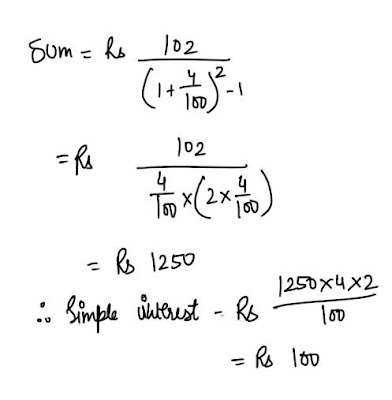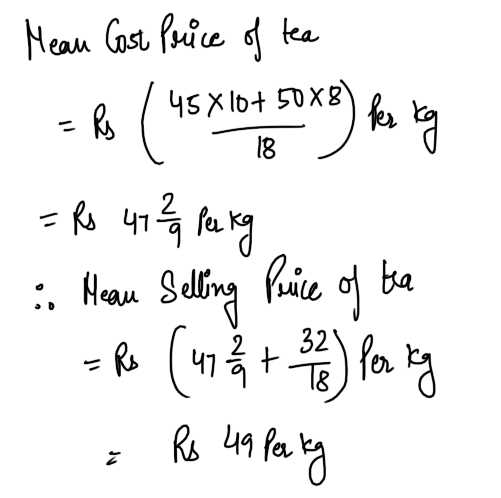New Students Offer - Use Code HELLO

# Mixed Aptitude Quiz for RBI Grade B exam - Part 2

#### Ques 1.

√484 + 3√729 + 9√512 equals
(a) 35
(b) 34
(c) 33
(d) None of these
Ans 1. Given  expression = 22 + 9 + 2 = 33

#### Ques 2

If the compound interest on a certain sum of money for 2 years at 4% p.a. is Rs 102, what is the simple interest ?
(a) Rs 101 1/4
(b) Rs 101
(c) Rs 100
(D) None of these
Ans 2.#### Ques 3.

Sumeet bought 10 kg of tea at Rs 45 per kg and 8 kg at Rs 50 per kg .He mixed both the brands and sold it at a total profit of Rs 32. What was the selling price per kg of the mixture ?
(a) Rs 48
(b) Rs 50
(c) Rs 49
(d) Rs 47
Ans 3.#### Ques 4.

A man sold a horse at 7% loss. He had sold it at 9% gain, he would have received Rs 32 more. What was the cost price ?
(a) Rs 215
(b) Rs 100
(c) Rs 150
(d) Rs 200
Ans 4.#### Ques 5.

A and B together can do a piece of work in a certain time. If they each did half the work separately , A would have to work one day less, B would have to work two days more. Find the time in which A and B together do the work.
(a) 4 days
(b) 7 days
(c) 8 days
(d) 5 days
Ans 5. Let the time taken by A and B to do the work together be x days.
A does half the work in  x- 1 days
or A does the work in 2 ( x - 1)  days.
B does half the work in x + 2 days.
or B does the work in 2 ( x + 2) days.#### Ques 6.

The ratio of boys to girls in a school is 5:2. The number of boys is more by 450 than that of the girls.How many students are there in that school ?
(a) 750
(b) 1050
(c) 300
(d) Cannot be determined
Ans 6. 5x- 2x = 450  =>  x = 150
Hence, total number of students in the school = 5x + 2x = 7x = 1050.

#### Ques 7.

Four years ago the ratio of Nitin's age to Manoj's age was 4 :7 .What will be the ratio of their ages now ?
(a) 8 : 11
(b) 6 : 9
(c) 4 : 7
(d) Cannot be determined
Ans 7. Nitin's age four years ago = 4x
Manoj's age  four years ago = 7x
Required ratio = 4x + 4/ 7x + 4 which cannot be determined.

#### Ques 8.

The average of 11numbers is 10.9. If the average of the first six is 10.5 and that of last six is 11.4, the middle number is
(a) 11.5
(b) 11.4
(c) 11.3
(d) None of these
Ans 8. Sum of 11 numbers = 11 x 10.9 = 119.9
Sum of first six numbers = 6 x 10.5 = 63.0
Sum of last six numbers = 6 x 11.4 = 68.4
. .The middle number = 68.4 + 63. 0 - 119.9 = 11.5

#### Ques 9.

The rate of interest at which Rs 1200 amounts to Rs 1323 in two years .when interest is compounded annually.is equal to
(a) 5%
(b) 10%
(c) 18%
(d) 4 %
Ans 9.#### Ques 10.

A mixture of 25 litres contains wine and water in the ratio 3 : 2 How much water should be added to the mixture to have wine and water in the ratio 1 : 1 ?
(a) 10 litres
(b) 5 litres
(c) 15 litres
(d) 12 litres
Ans 10.